# Accounting for Fatigue Data Scatter: Follow UpMay 29, 2015 By: Michael Bak

I recently wrote a blog entry on fatigue data scatter that discussed the fact that many published fatigue curves represent the average life, and based on the scatter, one should shift the fatigue curve to the specified probability of survival for that particular application.  Soon after, I received some excellent follow-up questions that I thought would be interesting to share.

Question 1:  For a given fatigue curve, such as those contained in Military Handbook 5 (MIL-HDBK-5), what probability of survival does the data represent?

Answer:  Unless specifically stated otherwise, MIL-HDBK-5 (and its replacement, the Metallic Materials Properties Development and Standardization or MMPDS) fatigue curves represent the typical or mean fatigue curves. For example, consider the fatigue curves for the 6061-T6 aluminum alloy, taken from MIL-HDBK-5 and shown below. Using these curves in a life prediction calculation would correspond to a probability of survival of 50%.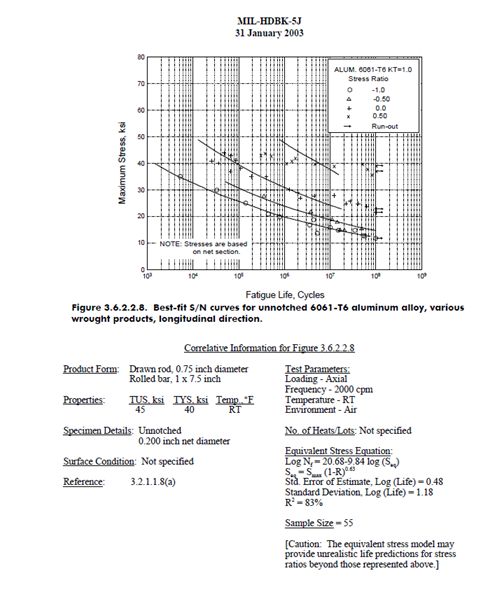Question 2:  Should the fatigue curves above be shifted to account for fatigue data scatter?

Answer:  Definitely! Unless it is acceptable for the fatigue life to be one failure out of two, the curve to use in fatigue life predictions should be shifted.

Question 3:  If the curve should be shifted, how is this done?

Answer:   The previous blog discussed that scatter is usually defined with a normal probability distribution that is either horizontal (Standard Error of Log10N) or vertical (Standard Error of Log10Stress).  The fatigue curve information should specify one of these.  For example, in the figure above, the Equivalent Stress Equation is given, which is the curve fit through the fatigue data.  The equation and its information is repeated here: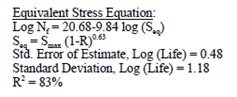The "Standard Deviation, Log(Life)" is equivalent to the Standard Error of Log10N.  So, the fatigue curve can be shifted horizontally using the expression from the previous blog: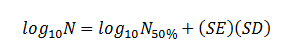where SE is equal to 1.18, and SD is the number of standard deviations to shift the curve to obtain the updated probability of survival.

It is often preferable to use the Standard Error of Log10Stress and shift the curve vertically. This is especially true if the fatigue curve has a horizontal flat section at high cycles that represents the endurance limit.  Shifting a curve left or right will not change the endurance limit, whereas shifting up and down will change the endurance limit, which represents more realistic behavior and is more conservative.  If the fatigue curve has a constant slope with respect to the log-log relationship, the Standard Error of Log10N and Standard Error of Log10Stress are related by the slope: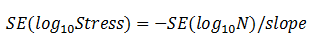Using the example above, the slope of the Equivalent Stress Equation is listed as -9.84. Therefore, the Standard Error of Log10Stress can be calculated: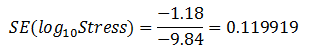As a way of verification, the ANSYS nCode fatigue module includes several built-in fatigue libraries, including one that has MIL-HDBK-5 materials.  For the 6061-T6 material data, which references the figure above, the following information is given: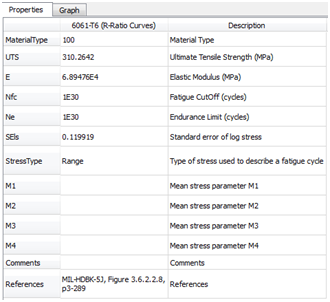Note that the Standard Error of Log10Stress = 0.119919, which is the same value we calculated above.

Question 4:  Are there other reasons to further shift the fatigue curve?

Answer:  In almost all cases, the answer is YES!  The current discussion only considers shifting the curve due to test data scatter.  But there are other reasons to further debit the fatigue curve:

• Fatigue tests using physically small test specimens are only a first indication of the real capability of the actual part.  Real parts are manufactured, machined and loaded in different ways.
• Surface condition has a significant effect on the fatigue life.  Note in the figure above that the surface condition is listed as "not specified".
• Fatigue tests require a large sample size to accurately describe the data scatter.  Many fatigue tests are run on only a small number of specimen (a sample size of 55 is listed for the aluminum material).

Based on these issues, an additional healthy factor of safety should be considered in addition to the fatigue data scatter.

These were great questions!  If anyone has additional questions, please let me know.